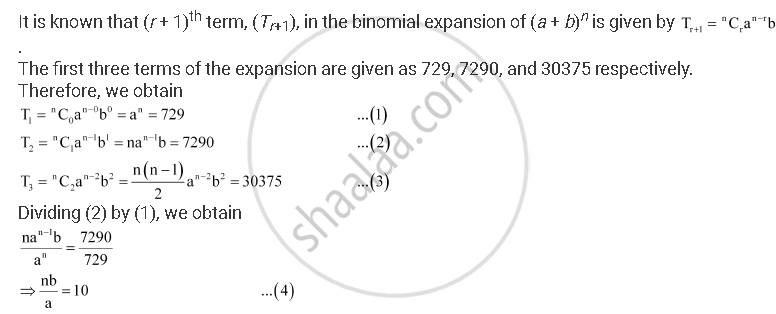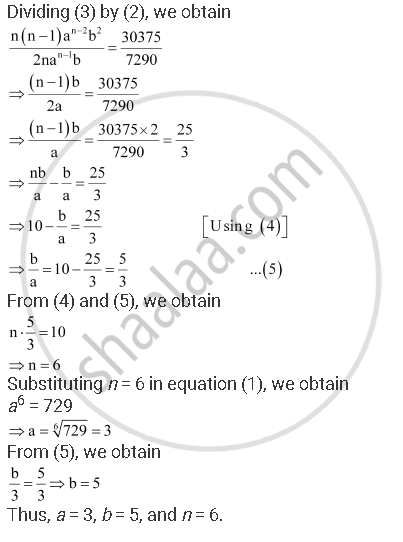# Find A, B and N in the Expansion of (A + B)N If the First Three Terms of the Expansion Are 729, 7290 and 30375, Respectively. - Mathematics

Find ab and n in the expansion of (a + b)n if the first three terms of the expansion are 729, 7290 and 30375, respectively.

#### SolutionConcept: Binomial Theorem for Positive Integral Indices
Is there an error in this question or solution?

#### APPEARS IN

NCERT Class 11 Mathematics
Chapter 8 Binomial Theorem
Q 1 | Page 175